Published on Feb 20,2019
25.8K Views
Email Post

## Backpropagation:

Backpropagation is a supervised learning algorithm, for training Multi-layer Perceptrons (Artificial Neural Networks).

I would recommend you to check out the following Deep Learning Certification blogs too:

But, some of you might be wondering why we need to train a Neural Network or what exactly is the meaning of training.

## Why We Need Backpropagation?

While designing a Neural Network, in the beginning, we initialize weights with some random values or any variable for that fact.

Now obviously, we are not superhuman. So, it’s not necessary that whatever weight values we have selected will be correct, or it fits our model the best.

Okay, fine, we have selected some weight values in the beginning, but our model output is way different than our actual output i.e. the error value is huge.

Now, how will you reduce the error?

Basically, what we need to do, we need to somehow explain the model to change the parameters (weights), such that error becomes minimum.

Let’s put it in an another way, we need to train our model.

One way to train our model is called as Backpropagation. Consider the diagram below: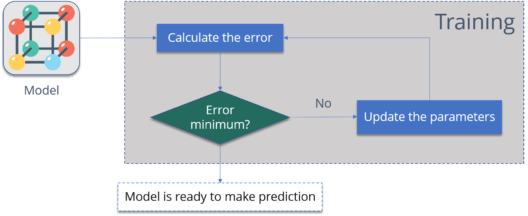Let me summarize the steps for you:

• Calculate the error – How far is your model output from the actual output.
• Minimum Error – Check whether the error is minimized or not.
• Update the parameters – If the error is huge then, update the parameters (weights and biases). After that again check the error. Repeat the process until the error becomes minimum.
• Model is ready to make a prediction – Once the error becomes minimum, you can feed some inputs to your model and it will produce the output.

I am pretty sure, now you know, why we need Backpropagation or why and what is the meaning of training a model.

Now is the correct time to understand what is Backpropagation.

## What is Backpropagation?

The Backpropagation algorithm looks for the minimum value of the error function in weight space using a technique called the delta rule or gradient descent. The weights that minimize the error function is then considered to be a solution to the learning problem.

Let’s understand how it works with an example:

You have a dataset, which has labels.

Consider the below table:

 Input Desired Output 0 0 1 2 2 4

Now the output of your model when ‘W” value is 3:

 Input Desired Output Model output (W=3) 0 0 0 1 2 3 2 4 6

Notice the difference between the actual output and the desired output:

 Input Desired Output Model output (W=3) Absolute Error Square Error 0 0 0 0 0 1 2 3 1 1 2 4 6 2 4

Let’s change the value of ‘W’. Notice the error when ‘W’ = ‘4’

 Input Desired Output Model output (W=3) Absolute Error Square Error Model output (W=4) Square Error 0 0 0 0 0 0 0 1 2 3 1 1 4 4 2 4 6 2 4 8 16

Now if you notice, when we increase the value of ‘W’ the error has increased. So, obviously there is no point in increasing the value of ‘W’ further. But, what happens if I decrease the value of ‘W’? Consider the table below:

 Input Desired Output Model output (W=3) Absolute Error Square Error Model output (W=2) Square Error 0 0 0 0 0 0 0 1 2 3 2 4 3 0 2 4 6 2 4 4 0

Now, what we did here:

• We first initialized some random value to ‘W’ and propagated forward.
• Then, we noticed that there is some error. To reduce that error, we propagated backwards and increased the value of ‘W’.
• After that, also we noticed that the error has increased. We came to know that, we can’t increase the ‘W’ value.
• So, we again propagated backwards and we decreased ‘W’ value.
• Now, we noticed that the error has reduced.

So, we are trying to get the value of weight such that the error becomes minimum. Basically, we need to figure out whether we need to increase or decrease the weight value. Once we know that, we keep on updating the weight value in that direction until error becomes minimum. You might reach a point, where if you further update the weight, the error will increase. At that time you need to stop, and that is your final weight value.

Consider the graph below: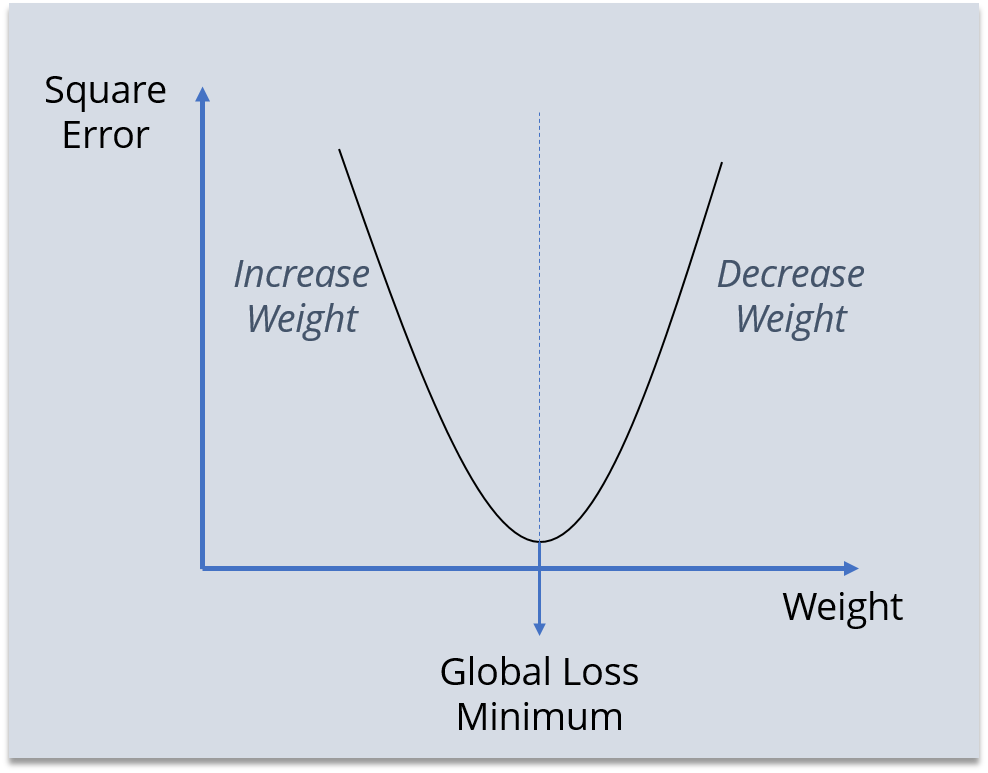We need to reach the ‘Global Loss Minimum’.

This is nothing but Backpropagation.

Let’s now understand the math behind Backpropagation.

## How Backpropagation Works?

Consider the below Neural Network: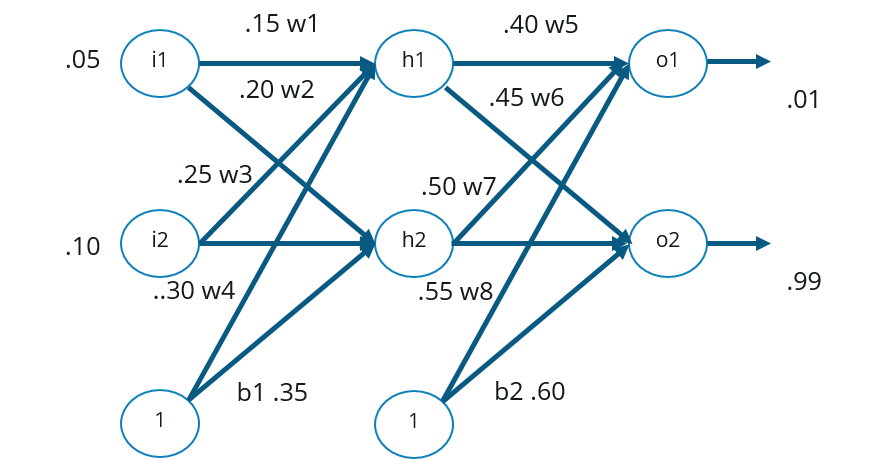The above network contains the following:

• two inputs
• two hidden neurons
• two output neurons
• two biases

Below are the steps involved in Backpropagation:

• Step – 1: Forward Propagation
• Step – 2: Backward Propagation
• Step – 3: Putting all the values together and calculating the updated weight value

### Step – 1: Forward Propagation

We will start by propagating forward.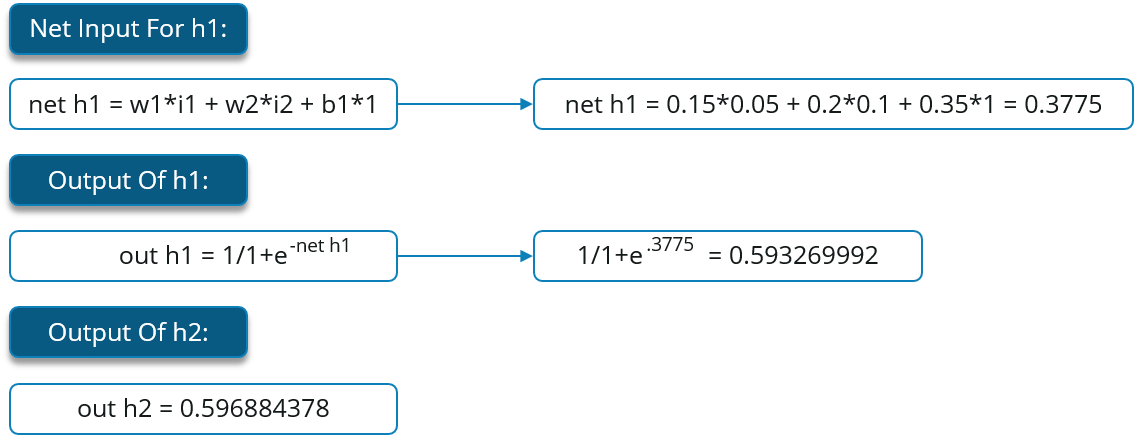We will repeat this process for the output layer neurons, using the output from the hidden layer neurons as inputs.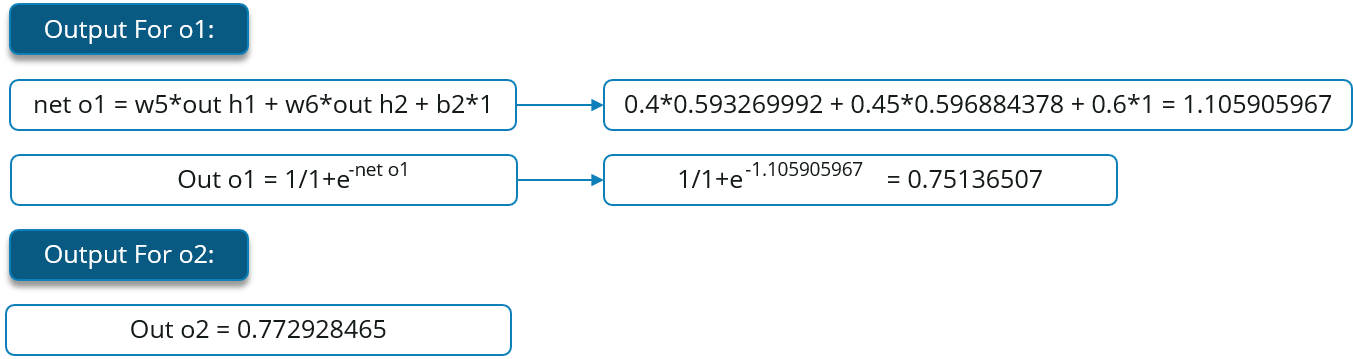Now, let’s see what is the value of the error: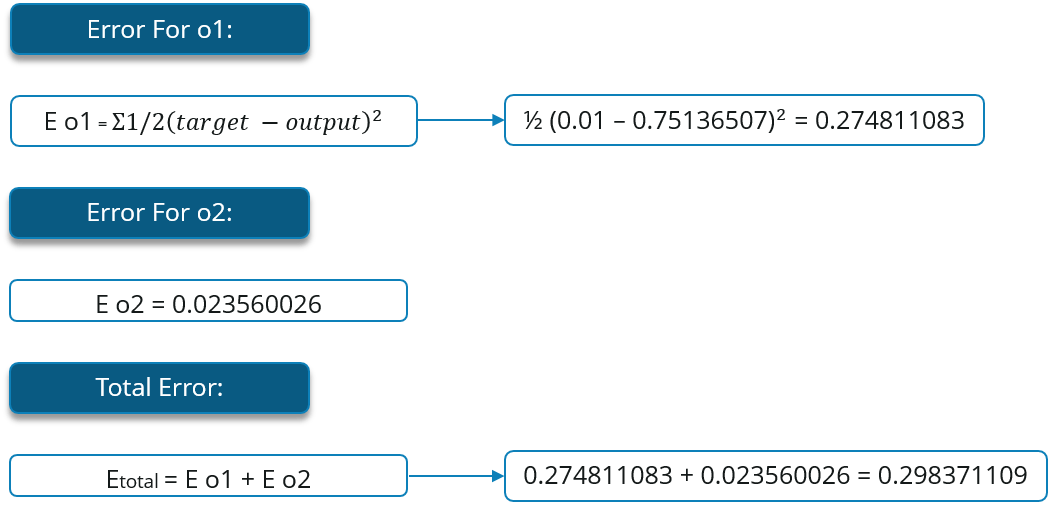### Step – 2: Backward Propagation

Now, we will propagate backwards. This way we will try to reduce the error by changing the values of weights and biases.

Consider W5, we will calculate the rate of change of error w.r.t change in weight W5.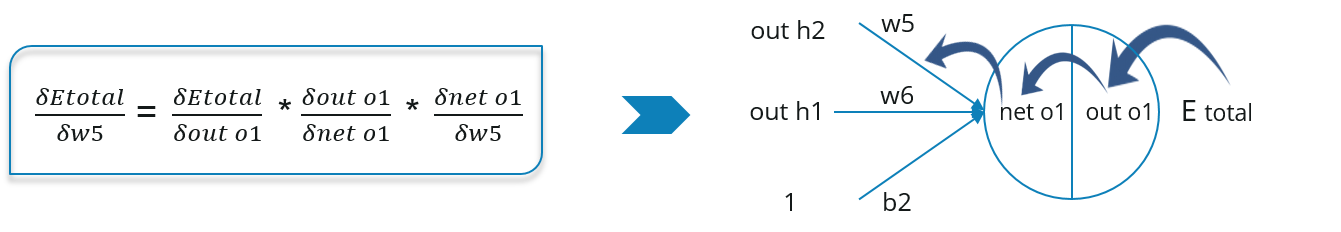Since we are propagating backwards, first thing we need to do is, calculate the change in total errors w.r.t the output O1 and O2.Now, we will propagate further backwards and calculate the change in output O1 w.r.t to its total net input.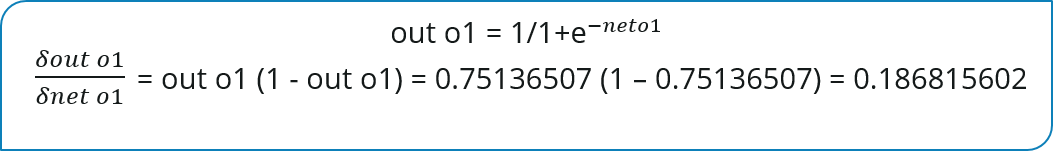Let’s see now how much does the total net input of O1 changes w.r.t  W5?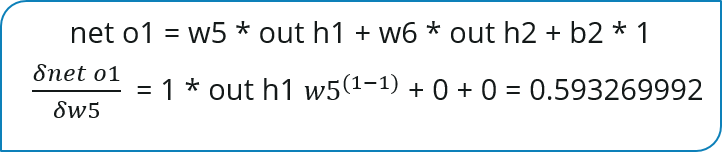### Step – 3: Putting all the values together and calculating the updated weight value

Now, let’s put all the values together:Let’s calculate the updated value of W5: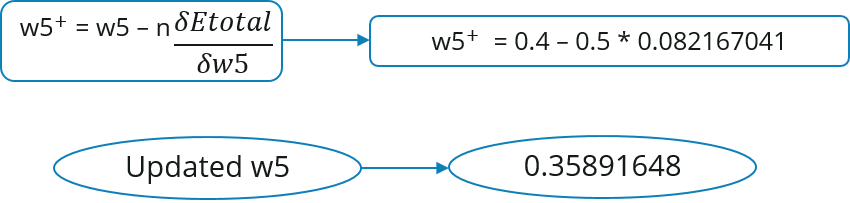• Similarly, we can calculate the other weight values as well.
• After that we will again propagate forward and calculate the output. Again, we will calculate the error.
• If the error is minimum we will stop right there, else we will again propagate backwards and update the weight values.
• This process will keep on repeating until error becomes minimum.

## Conclusion:

Well, if I have to conclude Backpropagation, the best option is to write pseudo code for the same.

### Backpropagation Algorithm:

```initialize network weights (often small random values)

do

forEach training example named ex

prediction = neural-net-output(network, ex)  // forward pass

actual = teacher-output(ex)

compute error (prediction - actual) at the output units

compute {\displaystyle \Delta w_{h}} for all weights from hidden layer to output layer  // backward pass

compute {\displaystyle \Delta w_{i}} for all weights from input layer to hidden layer   // backward pass continued

update network weights // input layer not modified by error estimate

until all examples classified correctly or another stopping criterion satisfied

return the network
```Trending Courses in this category

#### AI & Deep Learning with TensorFlow

5 (5450)
14k Learners Enrolled
Best Price 17,995 19,995

I hope you have enjoyed reading this blog on Backpropagation, check out the Deep Learning with TensorFlow Training by Edureka, a trusted online learning company with a network of more than 250,000 satisfied learners spread across the globe. The Edureka Deep Learning with TensorFlow Certification Training course helps learners become expert in training and optimizing basic and convolutional neural networks using real time projects and assignments along with concepts such as SoftMax function, Auto-encoder Neural Networks, Restricted Boltzmann Machine (RBM).

Got a question for us? Please mention it in the comments section and we will get back to you.